Courses

# Precipitation & Its Measurement Civil Engineering (CE) Notes | EduRev

## Civil Engineering (CE) : Precipitation & Its Measurement Civil Engineering (CE) Notes | EduRev

The document Precipitation & Its Measurement Civil Engineering (CE) Notes | EduRev is a part of the Civil Engineering (CE) Course Engineering Hydrology.
All you need of Civil Engineering (CE) at this link: Civil Engineering (CE)

Precipitation & General aspects of Hydrology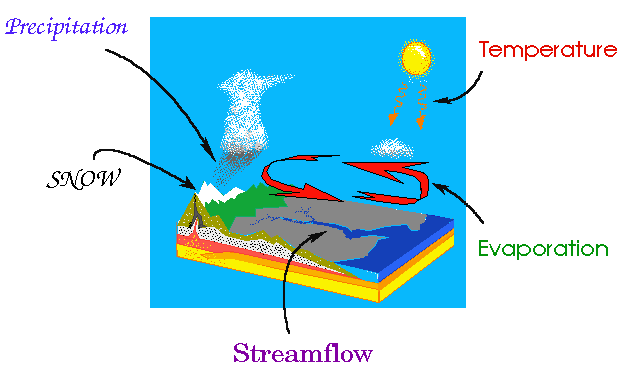1. Index of Wetness
(i) Index of wetness = (rainfall in a given year at a given place /average annual rainfall of that place) x 100
(ii) % Rain deficiency = 100 - % index of wetness
2. Aridity index
A.I = (PET - AET / PET) x 100
Where, A.I = Aridity index
PET = Potential Evapo- transpiration
AET = Actual Evapotranspiration
(i) AI ≤ 0 → Nonarid
(ii) 1 ≤ A.I ≤ 25 → Mild Arid
(iii) 26 ≤ A.I ≤ 50 → Moderate arid
(iv) A.I > 50 → Severe Arid
3. Optimum Number of rain Gauge: (N)
N = (CV / ∈)2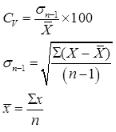where, Cv = Coefficient of variation,
∈ = Allowable % Error,
σ Standard deviation of the data, n = Number of stations,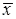mean of rainfall value
4. Estimation of missing rainfall data
(i)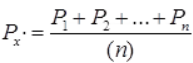If N1, N2…Nn < 10% of Nx
where, N1, N2,…Nx..Nn are normal annual precipitation of 1, 2,…x…n respectively.
P1, P2…Pn are rainfall at station 1, 2,…. N respectively.
And Px is the rainfall of station x.
Case: A minimum number of three stations closed to station ‘x’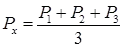(ii)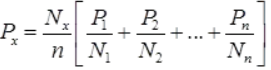If any of N1, N2, N3
Nn > 10% of N­x
5. Mean rainfall Data
To convert the point rainfall values at various into an average value over a catchment the following three methods are in use:
(i) Arithmetic Avg Method: when the rainfall measured at various stations in a catchment area is taken as the arithmetic mean of the station values.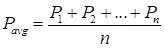Where, P1, P2…Pn are rainfall values
Of stations 1, 2…n respectively.
In practice this method is used very rerely.
(ii) Thiessen Polygon Method: in this method the rainfall recorded at each station is given a weightage on the basis of an area closest to the station.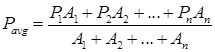Where, P1, P2…Pn are the rainfall data of areas A1, A2…An. The thiessen-polygon method of calculating the average precipitation over an area is superior to the arithmetic average method.
(iii) Isohyetal Method: An isohyet is a line joining points of equal rainfall magnitude. The recorded values for which areal average P is to be determined are then maked on the plot at appropirate stations. Neighbouring stations outside the catchment are also considered.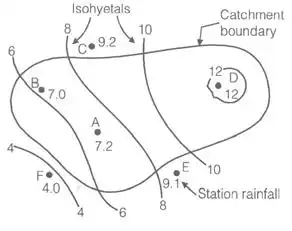Offer running on EduRev: Apply code STAYHOME200 to get INR 200 off on our premium plan EduRev Infinity!

## Engineering Hydrology

17 videos|21 docs|17 tests

,

,

,

,

,

,

,

,

,

,

,

,

,

,

,

,

,

,

,

,

,

;